Symbol
Problempiece. Answer the The following $or0b1emns$ below correspond to a $igsaw$ $Puzz\right)e$ $answerontne$ space provided. Direcacand find the $puzz|epiece$ Write the letter of your The width of a rectangle is The length of a rectangle is The $De\pi mete$ Scm shorter than its length. thrice its width. Its area is rectangle $is$ $42c\pi$ $80cm^{2}$ Faoinnf d d iia tts s the 75cm2. Find its $dimensi0ns$ area is Its area is $40cm^{2}$ Foinf d the $dimensi0ns$ length and width rectangle. $AnsVeT$ $AnsNer$ $AnsVyer$ The perimeter of a The width of a rectangle is The length of a rectangle is rectangle is 34mm and its 10cm shorter than its length. 9cm longer than its width. area is 70mm2. Find its Its area is 96 cm2. Find the Its area is 112cm2. Find the dimensions. length and width of the length and width of the rectangle. rectangle. $Answer:$ $|Answer$ Answer: The length of a rectangle is The length of a rectangle is six The perimeter a 8 cm longer than its width. its width. Its area is $216cm^{2}$ rectangle is 22 cm aonf d its Its area is $105cm^{2}$ Find the Find the length and width of the area is 30 cm. Find its length and width of the rectangle. dimensions. rectangle. $Answer$ $Answer^{°}$ $Answer$
7th-9th grade
Other
Search count: 120
SolutionQanda teacher - SHIVKUMARC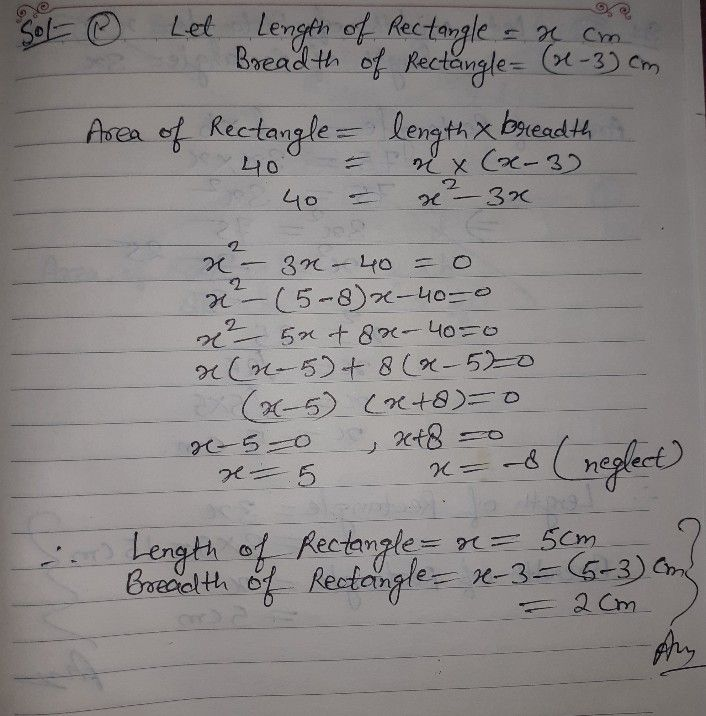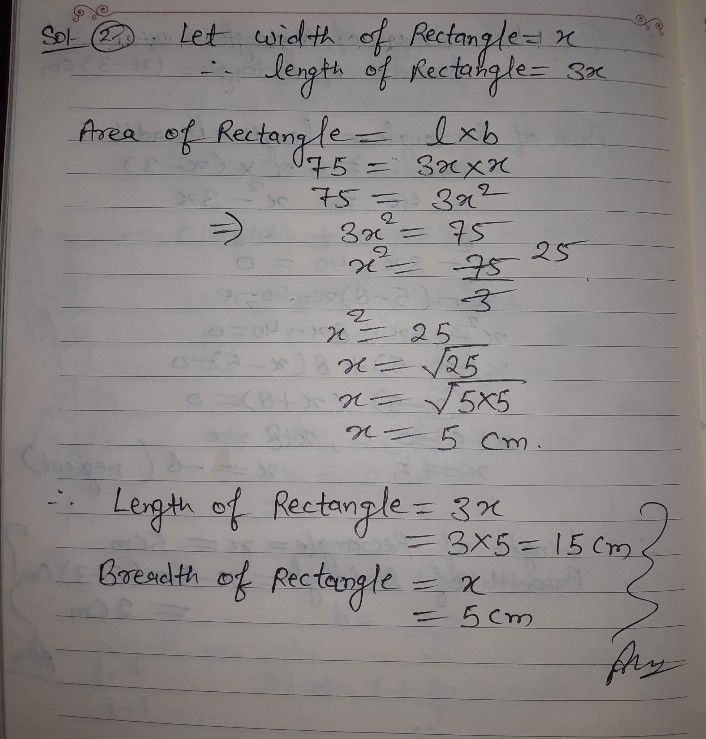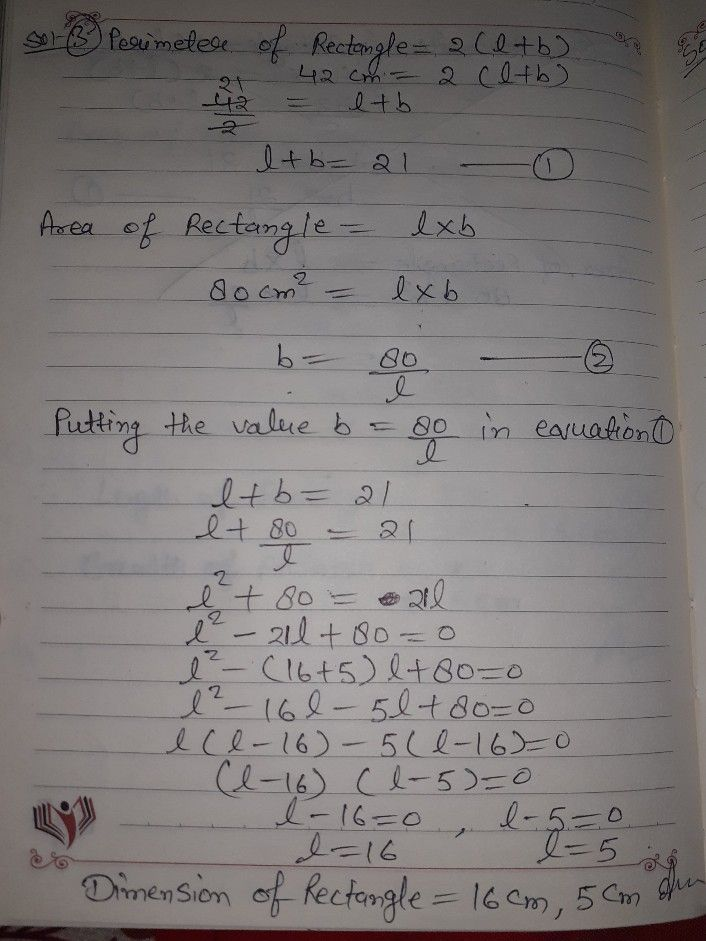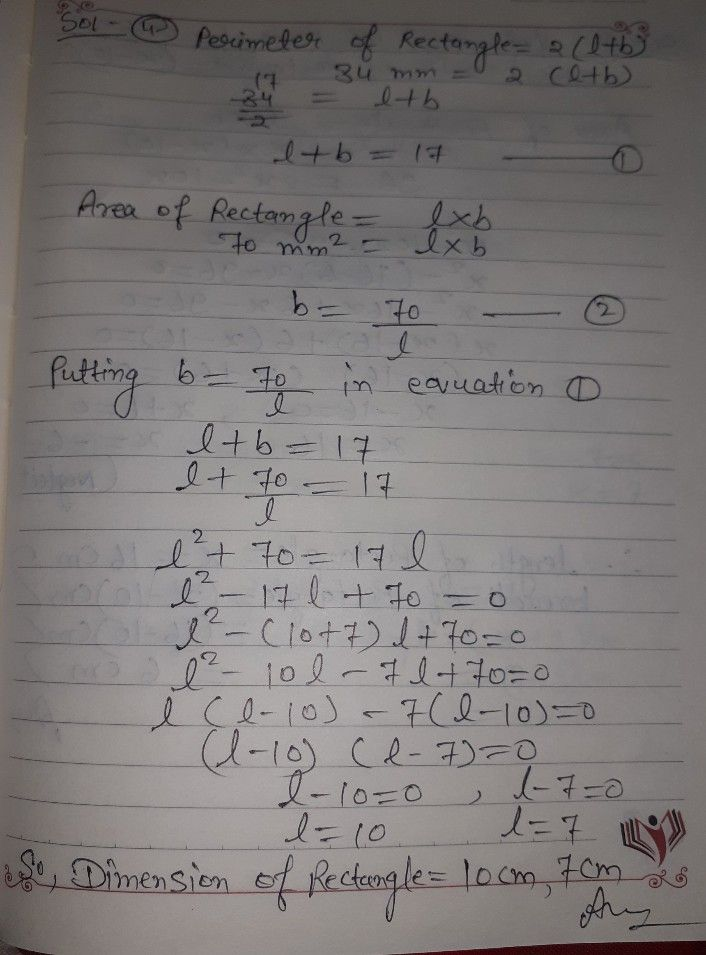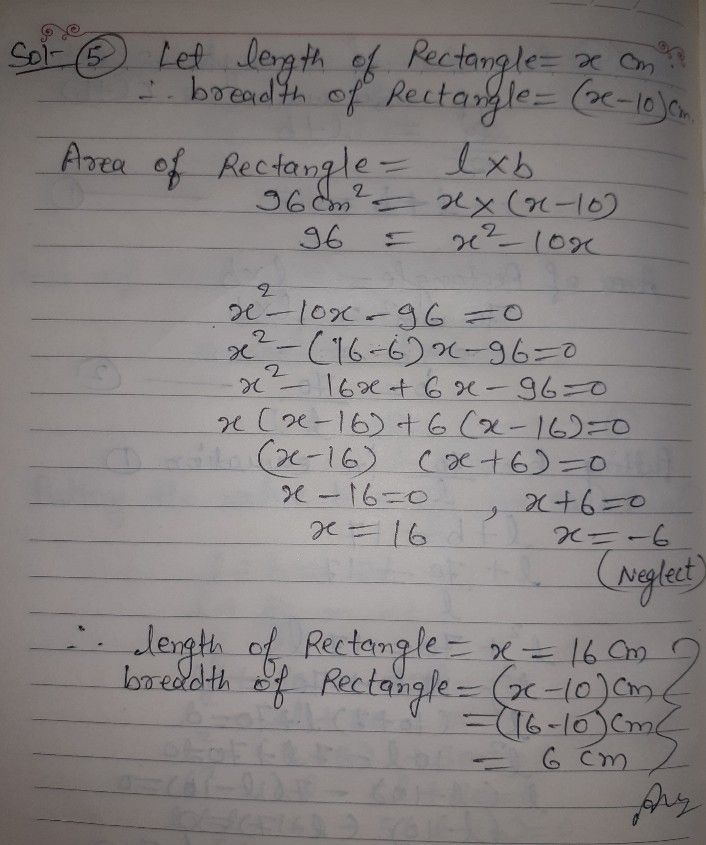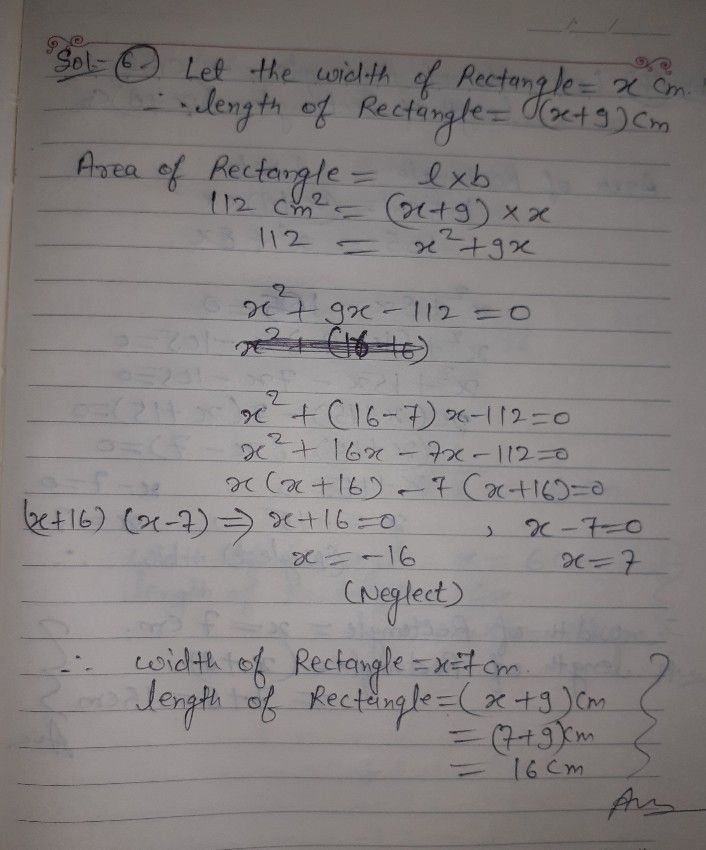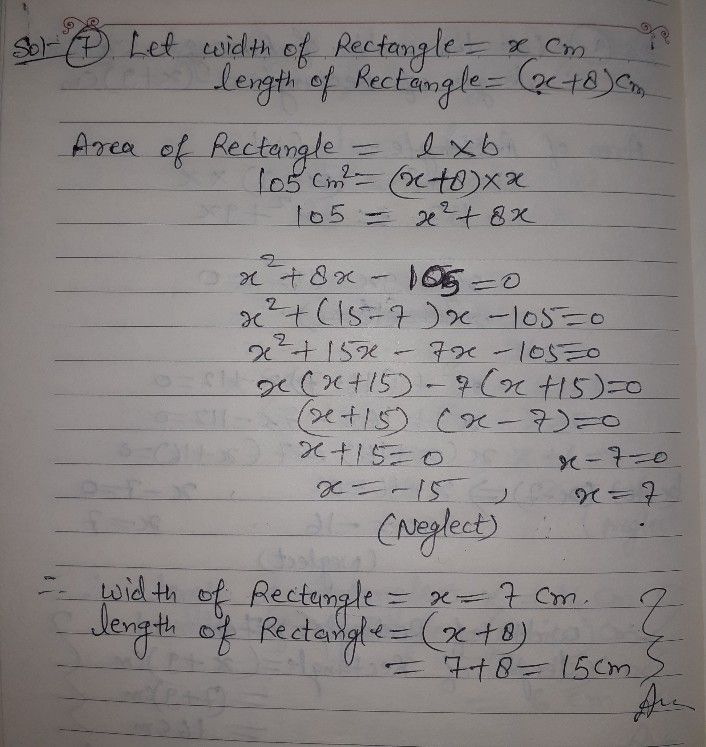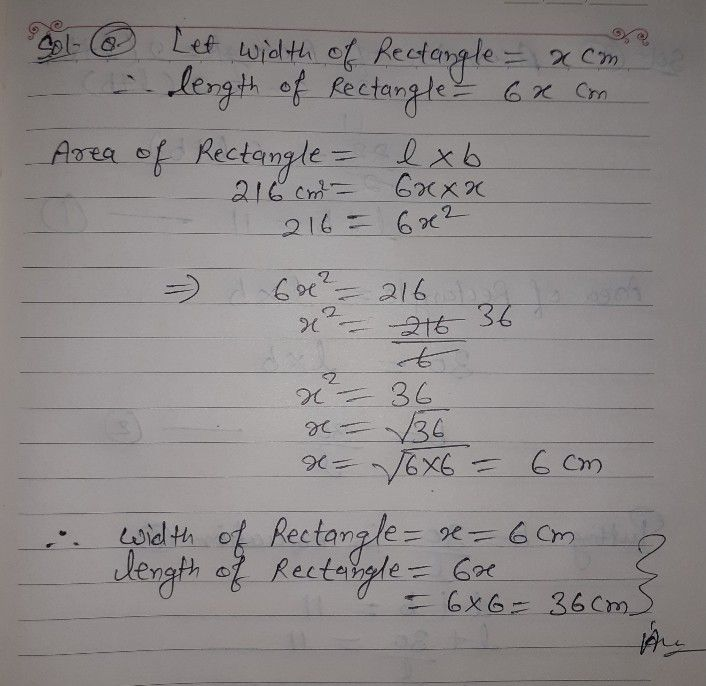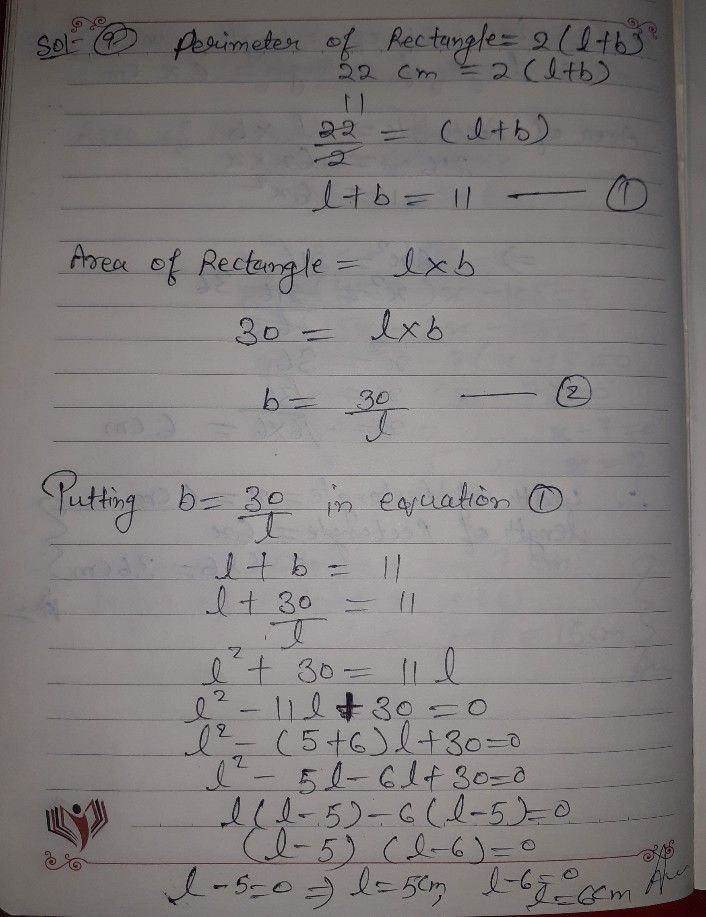okk
I hope you understand
If you have any problem with answer plz ask me or inform me first.
But If you're satisfied plz do like button and give 5 stars rating as a gift of Thank you...??Student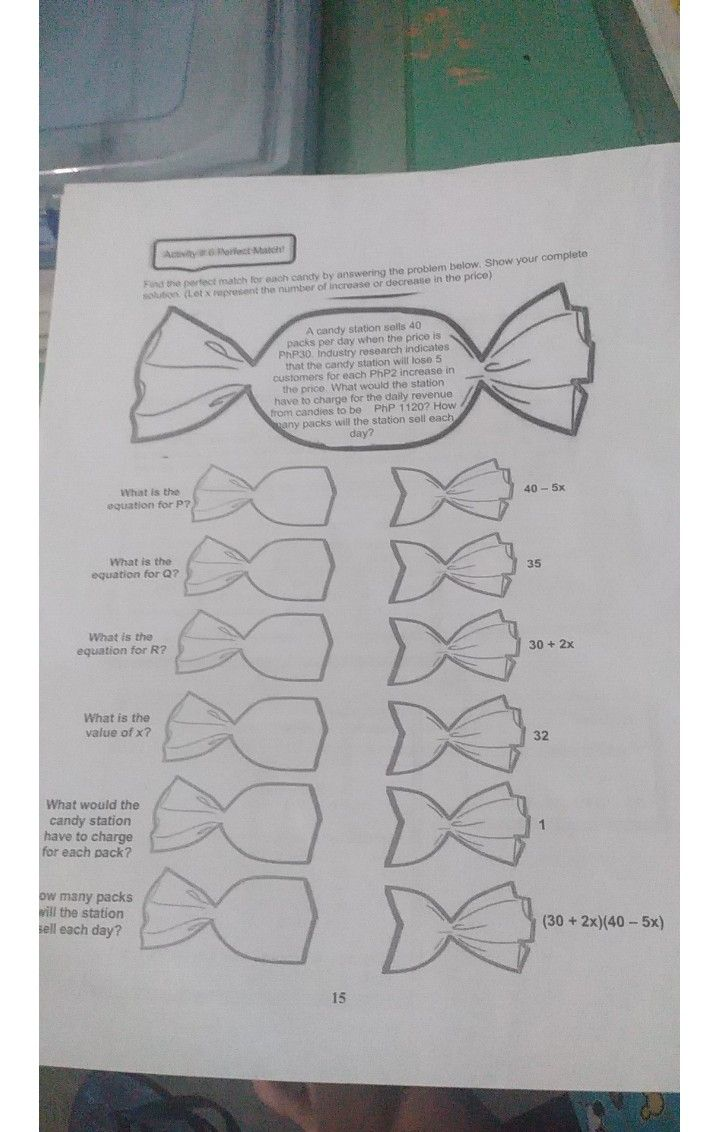please answer this
before im give the 5 star you
please answer thisQanda teacher - SHIVKUMARC
okk... but firstly you have to evaluate your first question.
and send this question in my preferred box.Student
i have solution thisQanda teacher - SHIVKUMARC
okk
plz evaluate your first question